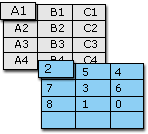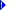# 在word表格中进行加减乘除法计算

在MicrosoftWord2003中，您可以对输入表格单元格的值进行加、减、乘、除运算。

在下面的示例中，每个表格单元格中的值用列字母和行号表示。例如，“A1”表示第一列和第一行中的单元格。执行下列步骤，将3加到单元格A1(A1的值为2)。

将插入点置于单元格A4中。

在“表格”菜单上，单击“公式”。

在“公式”下将自动显示公式(=Sum(Above))。

删除自动显示的公式，保留等号(=)。

在等号后输入(A1)+3。

单击“确定”。

公式的结果(2+3=5)将显示在单元格A4中。

在下列示例中执行上述步骤，然后在步骤4中插入下面的公式之一。加法

将数字加到单元格

=(A1)+3

在示例中等于5

对两个相邻的单元格求和

=SUM(A1:A2)

或

=(A1+A2)

在示例中等于9

对两个不相邻的单元格求和

=(A1+A3)

或

=(A1)+(A3)

在示例中等于10

对一组单元格求和

=SUM(B1:B3)

在示例中等于9减法

从单元格中减去数字

=(A1)-1

在示例中等于1

对两个单元格求差

=(A3-B2)

在示例中等于5乘法

将数字和单元格相乘

=(A1)*3

在示例中等于6

将两个相邻的单元格相乘

=PRODUCT(A1:A2)

在示例中等于14

将两个不相邻的单元格相乘

=(B1*B3)

或

=PRODUCT(B1)*(B3)

在示例中等于5除法

将数字和单元格相除

=(C2)/3

在示例中等于2

将两个单元格相除

=(C2)/(A1)

在示例中等于3

注意如果在表格中添加了行或列，则必须手动重新计算Word表格中的运算。要执行复杂的计算，您应当考虑使用MicrosoftExcel。